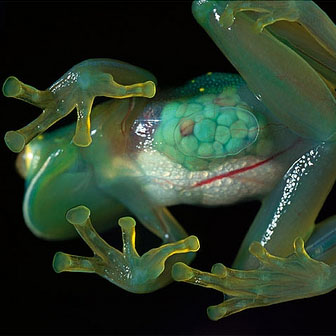# Deduce The Weight Of This FrogImage source: Mudfooted.com

A frog is jumping around a pond. At some time $t$, the frog is in the air and has a kinetic energy of $1~\mbox{J}$ and a momentum of magnitude $0.5~\mbox{kg}\cdot\mbox{m/s}$. What is the mass of the frog in kg?

×